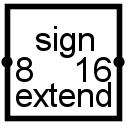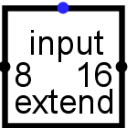#Bit Extender

Library: Wiring
Introduced: 2.5.0 (in Base library, moved to Wiring in 2.7.0)
Appearance:## Behavior

The bit extender transforms a value into a value of another bit width. If it's being transformed into a smaller bit width, it is simply truncated to keep the lowest-order bits. If it's being transformed into a large bit width, the lowest-order bits are the same, and you have a choice about what the additional high-order bits will be: They can all be 0, all be 1, all match the input's sign bit (its highest-order bit), or the component can have an additional one-bit input that determines the identity of these other bits.

## Pins

West edge:

The multi-bit input whose value is to be transformed. Bit width from Bit Width In attribute.

East edge:

The computed output. Bit width from Bit Width Out attribute.

North edge:

Specifies what the additional bits in the output should be. This pin is available only when the Extension Type attribute is Input. The bit width is 1.

## Attributes

When the component is selected or being added, the digits 0 through 9 alter the Bit Width In attribute and Alt-0 through Alt-9 alter its Bit Width Out attribute.

Bit Width In
The input's bit width.
Bit Width Out
The output's bit width.
Extension Type
Assuming the output bit width exceeds the input bit width, this attribute configures what the additional output bits should be. If Zero or One, the additional bits are 0 or 1 accordingly. If Sign, the additional bits are taken to match the highest-order bit in the input. And if Input, the component has a second input on its north side whose one-bit value is used for the additional bits.

None.

## Text Tool Behavior

None.

Back to Library Reference